# Pyramid

A pyramid is defined as a three-dimensional structure encompassing a polygon as its base. You must have heard about The Great Pyramid of Giza, which is structured in the same concept. Every corner of this structure is linked to a single apex which makes it appear as a distinct shape. The edge of each base and it’s top makes a triangle. In Maths, you will learn this geometrical shape and its properties along with the formulas for pyramids to find surface area and volumes.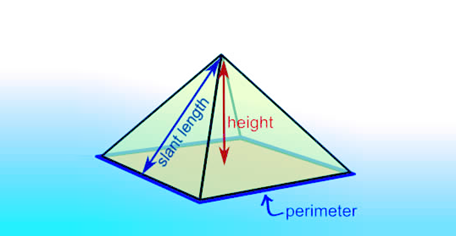## Types Of Pyramids

There are different types of pyramids that are named based on the shapes of their bases.

• Triangular Pyramid
• Square Pyramid
• Pentagonal Pyramid
• Right Pyramid
• Oblique Pyramid

### Triangular Pyramid

The base of this pyramid has the shape of a triangle; therefore, we call it as a triangular pyramid.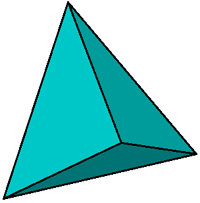### Square Pyramid

The base of this type of pyramid has a shape of a square; therefore, we call it a Square Pyramid.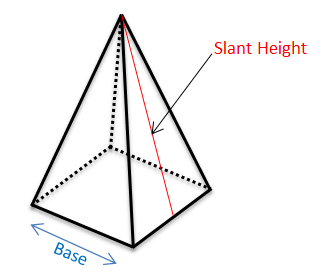### Pentagonal Pyramid

The base of this pyramid has the shape of a Pentagon; therefore, we call it a Pentagonal Pyramid.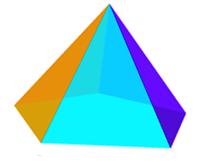### Right Pyramid

The apex of this pyramid is exactly over the middle of the base, hence named as Right Pyramid.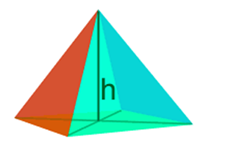### Oblique Pyramid

The apex of this pyramid is not exactly over the middle of its base and named as Oblique Pyramid.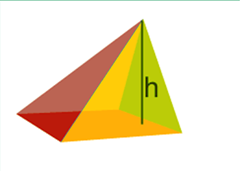### Regular vs Irregular Pyramid

To distinguish between regular and irregular Pyramid, you need to consider the shape of the base. If the base of a polygon is regular, it is labelled as Regular Pyramid, else it is considered as Irregular Pyramid. You can see the figures given below for both the pyramids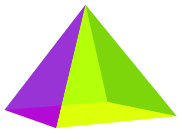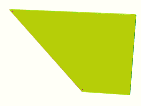Regular                                                                                             Irregular

## Pyramid Formulas

To find out the surface area and volumes of pyramids, some formulas can be used. The surface area of a pyramid is the total area of all the surfaces, including the base area, perimeter and slant height, such as;

Surface Area = (base area) + (1/2) × (perimeter) × (slant height)

To calculate the volume, the formula is;

Volume = 1/ 3 × (Base Area) × height

Note: The base area is the area of the base of the pyramid, which will vary based on its types.

Keep visiting us and download BYJU’S – The Learning App to continue learning more maths concepts in an engaging and effective way.

1. Nirmal hruday

Nice information you are giving byjus

2. Thamizh Iniyan

Super app